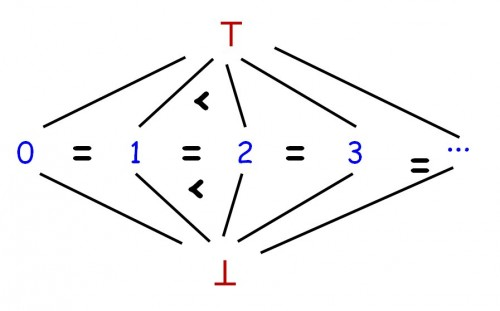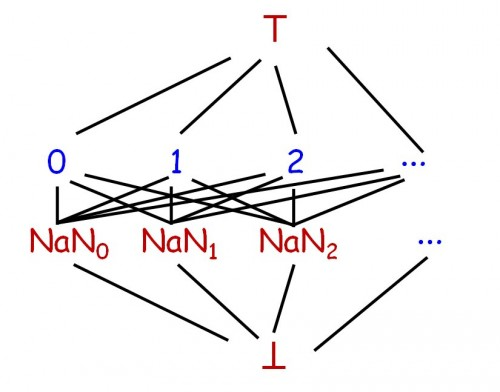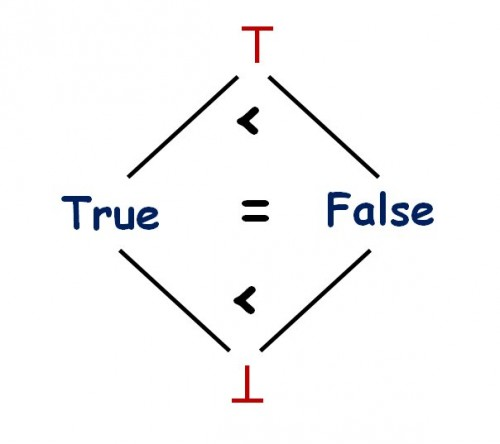Posts tagged ‘Numerics’

## Reflexivity, and other pillars of civilization

Let me start, dear reader of this blog, by probing your view of equality, and also of assignment. Two questions:

• Is a value x always equal to itself? (For the seasoned programmers in the audience: I am talking about a value, as in mathematics, not an expression, as in programming, which could have a side effect.)
• In programming, if we consider an assignment

x := y

and v is the value of y before that assignment (again, this little detour is to avoid bothering with side effects), is the value of x always equal to v after the assignment?

Maybe I should include here one of these Web polls that one finds on newspaper sites, so that you can vote and compare your answer to the Wisdom of Crowds. My own vote is clear: yes to both. Equality is reflexive (every value is equal to itself, at any longitude and temperature, no excuses and no exceptions); and the purpose of assignment is to make the value of the target equal to the value of the source. Such properties are some of the last ramparts of civilization. If they go away, what else is left?

#### 754 enters the picture

Now come floating-point numbers and the famous IEEE “754” floating-point standard . Because not all floating point operations yield a result usable as a floating-point number, the standard introduces a notion of “NaN”, Not a Number; certain operations involving floating-point numbers may yield a NaN. The term NaN does not denote a single value but a set of values, distinguished by their “payloads”.

Now assume that the value of x is a NaN. If you use a programming language that supports IEEE 754 (as they all do, I think, today) the test in

if x = x then …

is supposed to yield False. Yes, that is specified in the standard: NaN is never equal to NaN (even with the same payload); nor is it equal to anything else; the result of an equality comparison involving NaN will always be False.

Assignment behavior is consistent with this: if y (a variable, or an expression with no side effect) has a NaN value, then after

x := y

the test xy will yield False.

Before commenting further let me note the obvious: I am by no means a numerics expert; I know that IEEE 754 was a tremendous advance, and that it was designed by some of the best minds in the field, headed by Velvel Kahan who received a Turing Award in part for that success. So it is quite possible that I am about to bury myself in piles of ridicule. On the other hand I have also learned that (1) ridicule does not kill, so I am game; and more importantly (2) experts are often right but not always, and it is always proper to question their reasoning. So without fear let me not stop at the arguments that “the committee must have voted on this point and they obviously knew what they were doing” and “it is the standard and implemented on zillions of machines, you cannot change it now”. Both are true enough, but not an excuse to censor questions.

#### What are the criteria?

The question is: compatibility with an existing computer standard is great, but what about compatibility with a few hundred years of mathematics? Reflexivity of equality  is something that we expect for any data type, and it seems hard to justify that a value is not equal to itself. As to assignment, what good can it be if it does not make the target equal to the source value?

The question of assignment is particularly vivid in Eiffel because we express the expected abstract properties of programs in the form of contracts. For example, the following “setter” procedure may have a postcondition (expressed by the ensure clause):

set_x (v: REAL)
— Set the value of x (an attribute, also of type REAL) the value of v.
do
…
x := v
ensure
x = v
end

If you call this procedure with a NaN argument for a compiler that applies IEEE 754 semantics, and monitor contracts at run time for testing and debugging, the execution will report a contract violation. This is very difficult for a programmer to accept.

A typical example arises when you have an assignment to an item of an array of REAL values. Assume you are executing a [i] := x. In an object-oriented view of the world (as in Eiffel), this is considered simplified syntax  for the routine call a.put (x, i). The postcondition is that a [i] = x. It will be violated!

#### The experts’ view

I queried a number of experts on the topic. (This is the opportunity to express my gratitude to members of the IFIP working group 2.5 on numerical software , some of the world’s top experts in the field, for their willingness to respond quickly and with many insights.) A representative answer, from Stuart Feldman, was:

If I remember the debate correctly (many moons ago), NaN represents an indefinite value, so there is no reason to believe that the result of one calculation with unclear value should match that of another calculation with unclear value. (Different orders of infinity, different asymptotic approaches toward 0/0, etc.)

Absolutely correct! Only one little detail, though: this is an argument against using the value True as a result of the test; but it is not an argument for using the value False! The exact same argument can be used to assert that the result should not be False:

… there is no reason to believe that the result of one calculation with unclear value should not match that of another calculation with unclear value.

Just as convincing! Both arguments complement each other: there is no compelling reason for demanding that the values be equal; and there is no compelling argument either to demand that they be different. If you ignore one of the two sides, you are biased.

#### What do we do then?

The conclusion is not that the result should be False. The rational conclusion is that True and False are both unsatisfactory solutions. The reason is very simple: in a proper theory (I will sketch it below) the result of such a comparison should be some special undefined below; the same way that IEEE 754 extends the set of floating-point numbers with NaN, a satisfactory solution would extend the set of booleans with some NaB (Not a Boolean). But there is no NaB, probably because no one (understandably) wanted to bother, and also because being able to represent a value of type BOOLEAN on a single bit is, if not itself a pillar of civilization, one of the secrets of a happy life.

If both True and False are unsatisfactory solutions, we should use the one that is the “least” bad, according to some convincing criterion . That is not the attitude that 754 takes; it seems to consider (as illustrated by the justification cited above) that False is somehow less committing than True. But it is not! Stating that something is false is just as much of a commitment as stating that it is true. False is no closer to NaB than True is. A better criterion is: which of the two possibilities is going to be least damaging to time-honored assumptions embedded in mathematics? One of these assumptions is the reflexivity of equality:  come rain or shine, x is equal to itself. Its counterpart for programming is that after an assignment the target will be equal to the original value of the source. This applies to numbers, and it applies to a NaN as well.

Note that this argument does not address equality between different NaNs. The standard as it is states that a specific NaN, with a specific payload, is not equal to itself.

#### What do you think?

A few of us who had to examine the issue recently think that — whatever the standard says at the machine level — a programming language should support the venerable properties that equality is reflexive and that assignment yields equality.

Every programming language should decide this on its own; for Eiffel we think this should be the specification. Do you agree?

#### Some theory

For readers who like theory, here is a mathematical restatement of the observations above. There is nothing fundamentally new in this section, so if you do not like strange symbols you can stop here.

The math helps explain the earlier observation that neither True nor False is more“committing” than the other. A standard technique (coming from denotational semantics) for dealing with undefinedness in basic data types, is to extend every data type into a lattice, with a partial order relation meaning “less defined than”. The lattice includes a bottom element, traditionally written “” (pronounced “Bottom”) and a top element written (“Top”). represents an unknown value (not enough information) and an error value (too much information). Pictorially, the lattice for natural numbers would look like this:The lattice of integers

On basic types, we always use very simple lattices of this form, with three kinds of element: , less than every other element; , larger than all other elements; and in-between all the normal values of the type, which for the partial order of interest are all equal. (No, this is not a new math in which all integers are equal. The order in question simply means “is less defined than”. Every integer is as defined as all other integers, more defined than , and less defined than .) Such lattices are not very exciting, but they serve as a starting point; lattices with more interesting structures are those applying to functions on such spaces — including functions of functions —, which represent programs.

The modeling of floating-point numbers with NaN involves such a lattice; introducing NaN means introducing a value. (Actually, one might prefer to interpret NaN as , but the reasoning transposes immediately.)  More accurately, since there are many NaN values, the lattice will look more like this:The lattice of floats in IEEE 754

For simplicity we can ignore the variety of NaNs and consider a single .

Functions on lattices — as implemented by programs — should satisfy a fundamental property: monotonicity. A function f  is monotone (as in ordinary analysis) if, whenever xy, then f (x) ≤ f (y). Monotonicity is a common-sense requirement: we cannot get more information from less information. If we know less about x than about y, we cannot expect that any function (with a computer, any program) f will, out of nowhere, restore the missing information.

Demanding monotonicity of all floating-point operations reflects this exigency of monotonicity: indeed, in IEEE 754, any arithmetic operation — addition, multiplication … — that has a NaN as one of its arguments must yield a Nan as its result. Great. Now for soundness we should also have such a property for boolean-valued operations, such as equality. If we had a NaB as the  of booleans, just like NaN is the  of floating-point numbers,  then the result of NaN = NaN would be NaB. But the world is not perfect and the IEEE 754 standard does not govern booleans. Somehow (I think) the designers thought of False as somehow less defined than True. But it is not! False is just as defined as True in the very simple lattice of booleans; according to the partial order, True and False are equal for the relevant partial order:The lattice of booleans

Because any solution that cannot use a NaB will violate monotonicity and hence will be imperfect, we must resort to heuristic criteria. A very strong criterion in favor of choosing True is reflexivity — remaining compatible with a fundamental property of equality. I do not know of any argument for choosing False.

#### The Spartan approach

There is, by the way, a technique that accepts booleans as we love them (with two values and no NaB) and achieves mathematical rigor. If operations involving NaNs  truly give us pimples, we can make any such operation trigger an exception. In the absence of values,  this is an acceptable programming technique for representing undefinedness. The downside, of course, is that just about everywhere the program must be ready to handle the exception in some way.

It is unlikely that in practice many people would be comfortable with such a solution.

#### Final observations

Let me point out two objections that I have seen. Van Snyder wrote:

NaN is not part of the set of floating-point numbers, so it doesn’t behave as if “bottom” were added to the set.

Interesting point, but, in my opinion not applicable: is indeed not part of the mathematical set of floating point numbers, but in the form of NaN it is part of the corresponding type (float in C, REAL in Eiffel); and the operations of the type are applicable to all values, including NaN if, as noted, we have not taken the extreme step of triggering an exception every time an operation uses a NaN as one of its operands. So we cannot free ourselves from the monotonicity concern by just a sleight of hands.

Another comment, also by Van Snyder (slightly abridged):

Think of [the status of NaN] as a variety of dynamic run-time typing. With static typing, if  x is an integer variable and y

x := y

x = y

True; and a compelling argument to require that conversions satisfy equality as a postcondition! Such  reasoning — reflexivity again — was essential in the design of the Eiffel conversion mechanism , which makes it possible to define conversions between various data types (not just integers and reals and the other classical examples, but also any other user types as long as the conversion does not conflict with inheritance). The same conversion rules apply to assignment and equality, so that yes, whenever the assignment x := y is permitted, including when it involves a conversion, the property x = y  is afterwards always guaranteed to hold.

It is rather dangerous indeed to depart from the fundamental laws of mathematics.

#### References

 IEEE floating-point standard, 754-2008; see summary and references in the Wikipedia entry.